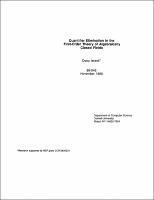## Quantifier Elimination in the First-Order Theory of Algebraically Closed Fields##### Abstract
We consider the problem of deciding whether a set of multivariate polynomials with coefficients in any field $F$ have a common algebraic solution. In this paper we develop a fast parallel algorithm for solving this decision problem. Since the proposed algorithm is algebraic, it easily yields a procedure for quantifier elimination in the theory of an arbitrary algebraically closed field. More precisely, we show how to decide whether $m$ polynomials in $n$ variables, each of degree at most $d$, with coefficients in an arbitrary field $F$ have a common zero in the algebraic closure of $F$, using sequential time $m^{n + O(1)} d^{n^{2} + O(n)}$, or parallel time $O(n^{3} \log^{3} d \log m)$ with $m^{n + O(1)} d^{n^{2} + O(n)}$ processors, in the operations of the coefficient field $F$. Using randomization, this may be improved to $m^{O(1)} d^{O(n)}$ time. In addition, the construction is used give a direct EXSPACE algorithm for quantifier elimination in the theory of an algebraically-closed field, which runs in PSPACE or parallel polynomial time when restricted to formulas with a fixed number of alternations of quantifiers.
1988-11
##### Publisher
Cornell University
##### Keywords
computer science; technical report
##### Previously Published As
http://techreports.library.cornell.edu:8081/Dienst/UI/1.0/Display/cul.cs/TR88-948
technical report